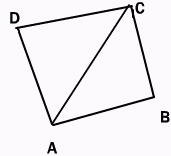Name: ___________________Date:___________________

kwizNET Subscribers, please login to turn off the Ads!
 Email us to get an instant 20% discount on highly effective K-12 Math & English kwizNET Programs!

### High School Mathematics - 29.20 Area of Quadrilateral

 The area of quadrilateral can be found by splitting it into two triangles and ading up their areas. The area of the quadrilateral whose vertices taken in order are A(x1, y1), B(x2, y2), C(x3, y3), D(x4, y4).Write down the coordinates as shownArea of Quad ABCD = Area of triangle ABC + Area of triangle ACD = 1/2{(x1y2)-(x2y1+(x2y3-x3y2(x3y1-x1y3)} + 1/2{(x1y3-x3y1)+x3y4-x4y3)+ (x4y1-x1y4} =1/2[(x1y2-x2y1)+(x2y3-x3y2)+(x3y4-x4y3)+(x4y1-x1y4)] Example: Find the area of the quadrilateral whose vertices are (3.4), (0,5),(2,-1) and (3,-2).The required area is 1/2[3x5-0x4+0x(-1)-5x2+2x(-2)-(-1)(3)+3x4-(-2)(3)] Answer: 11 sq.units Directions: Solve the following.Name: ___________________Date:___________________

### High School Mathematics - 29.20 Area of Quadrilateral

 Q 1: Find the area of the quadrilateral whose vertices are (1,1), (3,4), (5,-2) and (4,-7).41/235/239/2 Q 2: Find the area of the quadrilateral whose vertices are (1,1), (7,-3), (12,2) and (7,21).122112132 Q 3: If the area of the quadrilateral whose angular points taken in order are (1,2), (-5,6), (7,4) and (h,-2), find h.536 Q 4: Find the area of the quadrilateral whose vertices are (1,1), (5,-2), (3,4) and (4,-7).201820 1/2 Question 5: This question is available to subscribers only! Question 6: This question is available to subscribers only!

#### Subscription to kwizNET Learning System costs less than \$1 per month & offers the following benefits:

• Unrestricted access to grade appropriate lessons, quizzes, & printable worksheets
• Instant scoring of online quizzes
• Progress tracking and award certificates to keep your student motivated
• Unlimited practice with auto-generated 'WIZ MATH' quizzes
• Child-friendly website with no advertisements

© 2003-2007 kwizNET Learning System LLC. All rights reserved. This material may not be reproduced, displayed, modified or distributed without the express prior written permission of the copyright holder. For permission, contact info@kwizNET.com
For unlimited printable worksheets & more, go to http://www.kwizNET.com.